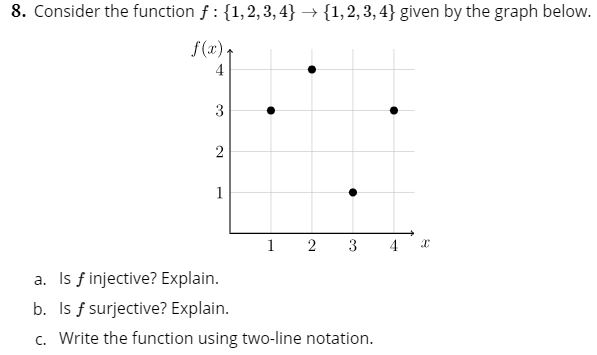# 8. Consider the function f 1,2,3,41,2,3,4} given by the graph below.f(x)4321234a. Is f injective? Explain.b. Is f surjective? Explain.c. Write the function using two-line notation.

Question
8 viewshelp_outlineImage Transcriptionclose8. Consider the function f 1,2,3,4 1,2,3,4} given by the graph below. f(x) 4 3 2 1 2 3 4 a. Is f injective? Explain. b. Is f surjective? Explain. c. Write the function using two-line notation. fullscreen
check_circle

star
star
star
star
star
1 Rating
Step 1

F:{1,2,3,4}->{1,2,3,4}

From the given graph it can be said that:

F(1) = 3

F(2) = 4

F(3) = 1

F(4) = 3

Since, the given function f is neither injective nor surjective because injective function always maps distinct elemen...

### Want to see the full answer?

See Solution

#### Want to see this answer and more?

Solutions are written by subject experts who are available 24/7. Questions are typically answered within 1 hour.*

See Solution
*Response times may vary by subject and question.
Tagged in

### Math